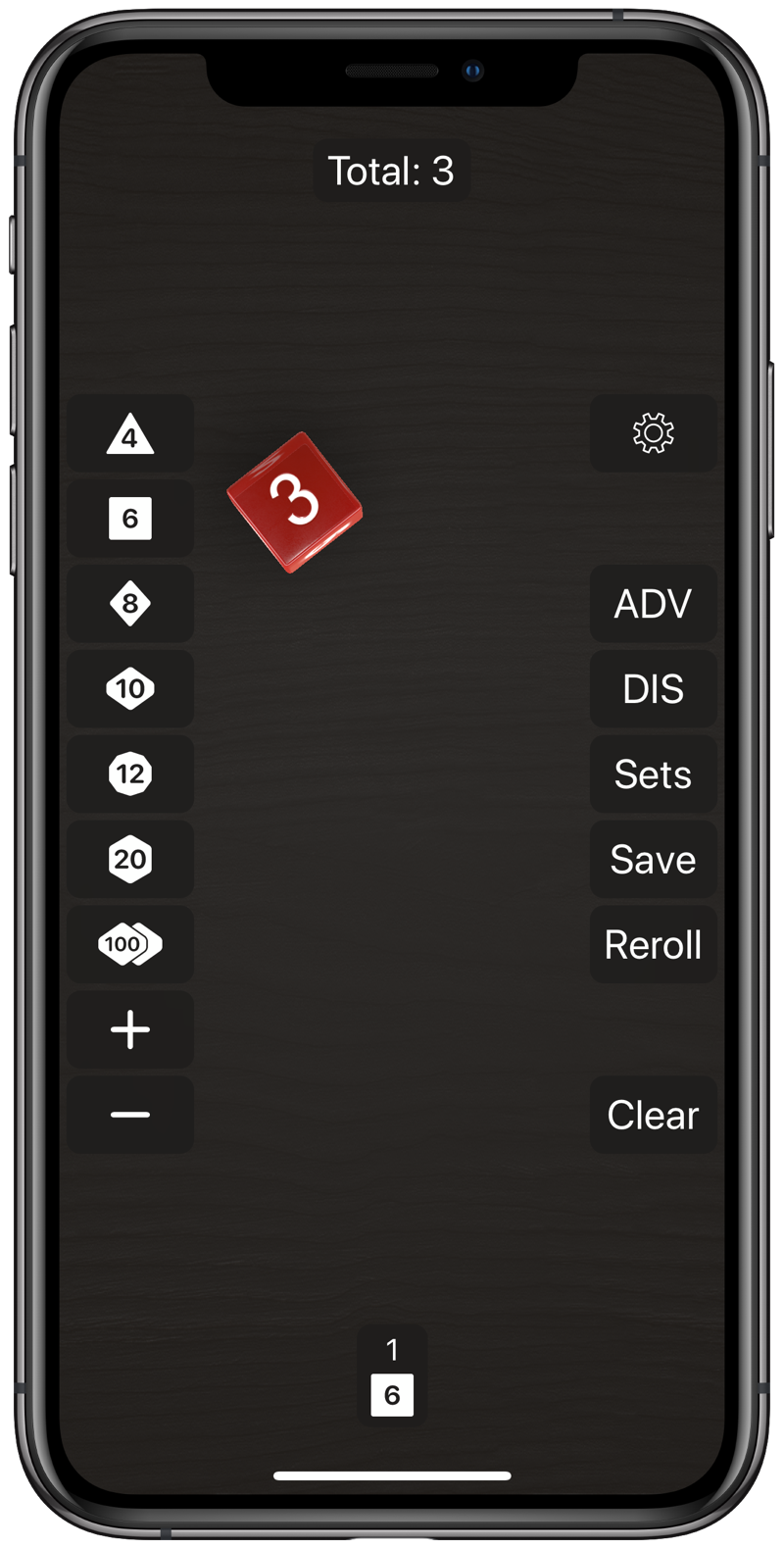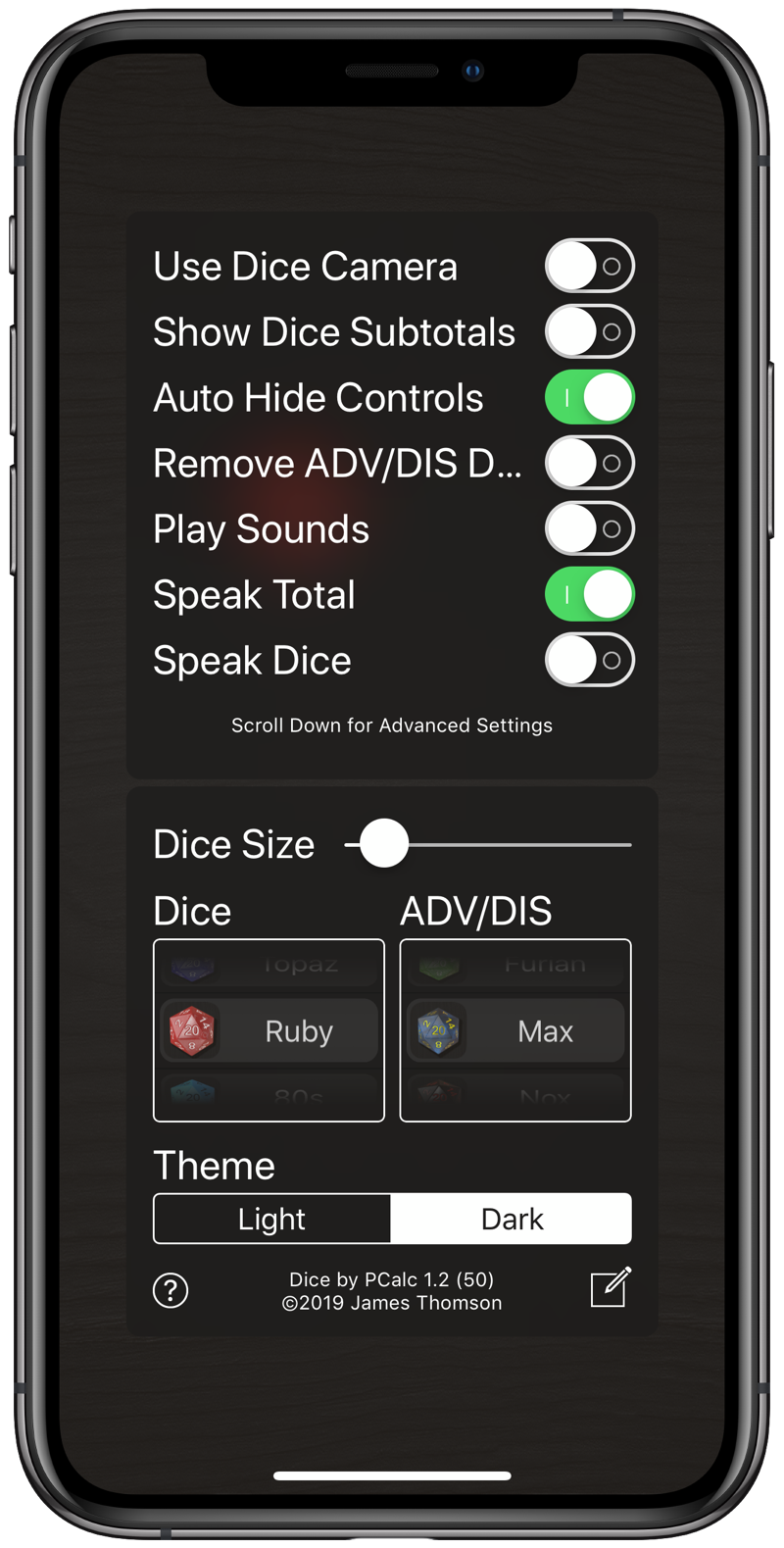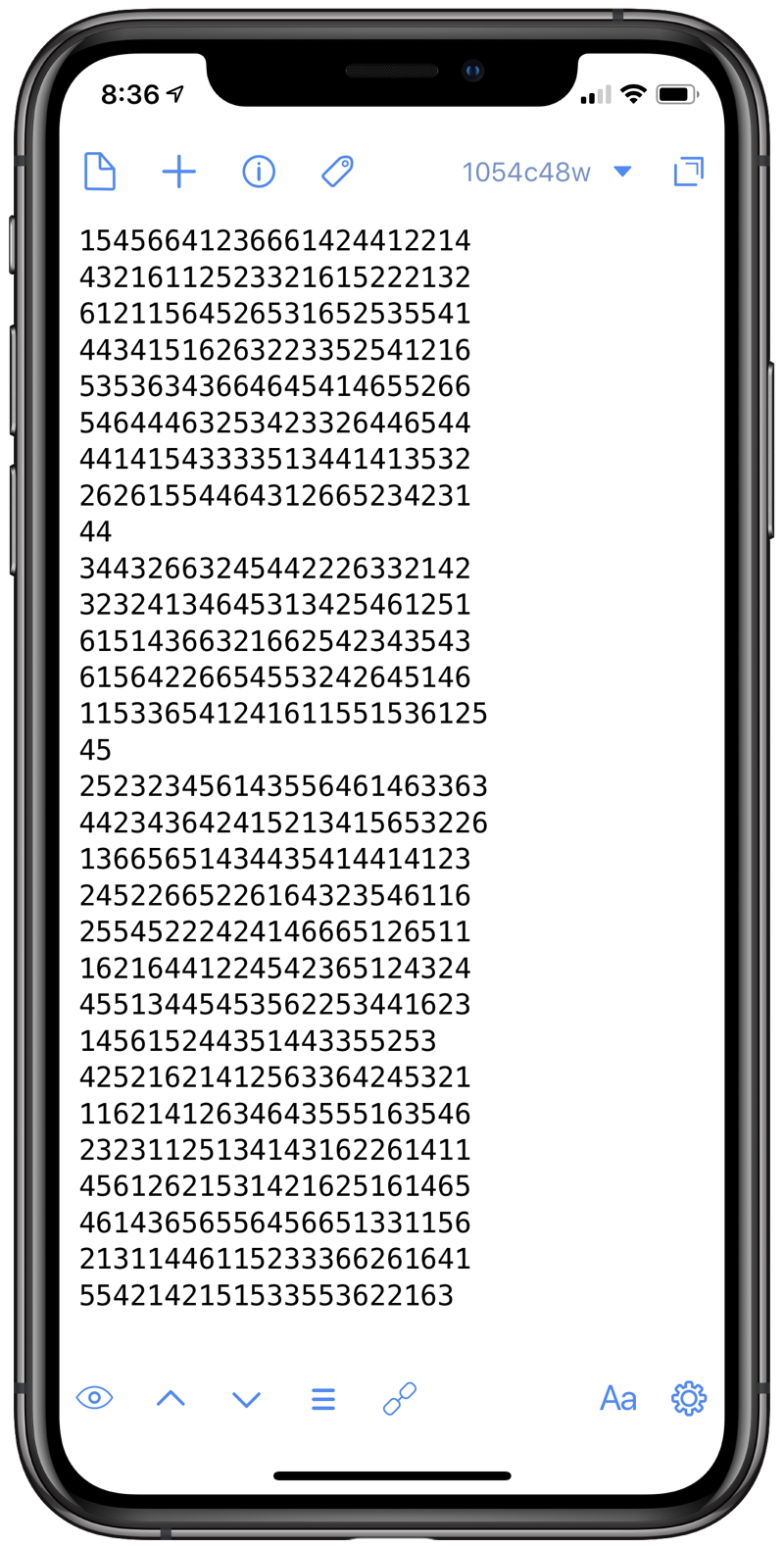# Gonna Roll the Bones

Early last month, James Thomson released a new iOS dice-rolling app called, with an eye to the App Store’s search function, Dice by PCalc. Being James, he didn’t just hook up a random number generator to an animation of dice, he used a physics engine to simulate the mechanics of rolling dice.My first thought was “how random will this simulation be?” What we think of as randomness in dice-rolling and coin-tossing is really based on the chaos inherent in the dynamics of these actions. A coin flip, for example, is a completely deterministic process and seems random to us (and can pass statistical tests) only because the results change significantly due to small changes in the initial conditions.1 There’s a great Numberphile video in which Persi Diaconis discusses this. In fact, you should just watch all the Diaconis videos, including the two on fair dice.

So my question was really about how good the physics engine was at simulating real chaotic dynamic processes. Would the rolls that come out of Dice pass the kind of statisical tests that rolls of fair physical dice would?

The best way to check this would be to generate a bunch of rolls in Dice and then run a statistical test on the results. Here is where my laziness kicked in. Sure, I was interested in this, but was I interested enough to do all the tedious work necessary to collect the data?

Yes and no. I certainly wasn’t going to roll with Dice and then type in the number that came up. Even with two iOS devices running in parallel, one for the rolling and one for the typing, that was too painful to contemplate.

I then thought about dictating the numbers. I’ve had success dictating measurements while I’m working in the lab. But then I realized I didn’t have to do the dictation myself.

Dice has a setting for speaking the results. By turning that on, I could put my iPad and iPhone near each other and have Dice running on the iPad dictating its results to Drafts running on the iPhone.I figured Drafts was the best app to dictate into because it’s more forgiving of pauses than other apps and there were definitely gaps between rolls. Even so, Drafts would typically time out after 20–25 rolls, so I got in the habit of stopping dictation when the line of numerals got to that length.

By continually tapping Dice’s Reroll button, I soon had a list of about 1000 rolls (1005, to be precise) of a single six-sided die collected in Drafts.(The short lines came when I mistapped on one of the two devices and I had to restart the dictation on a new line.)

Now it was time to analyze the data. First, I cleaned up the data by searching for all the newline characters and deleting them. That gave me one long string of numerals that I could paste into my Python analysis script.

The purpose of the script is to count all the occurrences of each number. We can then use the chi-square test to see if the counts are close enough to equal to be considered uniformly distributed.

Here’s the script (where I’ve broken up the dice string to make it easy to read):

python:
1:  from collections import Counter
2:
3:  dice = '62331245646253365416252416456666441662363644345422542256142\
4:  1466414261214312335455454662646535364552643553665562651445113223516\
5:  4345133236466256615163133461424555341161364531342162154345456123551\
6:  5423652314323336623453164254465211353346441264444255242555423541323\
7:  6533463525333334261214625566242633555332152324134625433336551162653\
8:  6315124456213426444412453433411545664123666142441221443216112523321\
9:  6152221326121156452653165253554144341516263223352541216535363436646\
10:  4541465526654644463253423326446544441415433335134414135322626155446\
11:  4312665234231443443266324544222633214232324134645313425461251615143\
12:  6632166254234354361564226654553242645146115336541241611551536125452\
13:  5232345614355646146336344234364241521341565322613665651434435414414\
14:  1232452266522616432354611625545222424146665126511162164412245423651\
15:  2432445513445453562253441623145615244351443355253425216214125633642\
16:  4532111621412634643555163546232311251341431622614114561262153142162\
17:  5161465461436565564566513311562131144611523336626164155421421515335\
18:  53622163'
19:
20:  n = len(dice)
21:  m = n/6
22:  x = Counter(dice)
23:
24:  chi2 = 0
25:  for i in range(1, 7):
26:    k = str(i)
27:    x2i = (x[k] - m)**2/m
28:    print(f'{k}:  {x2i:.4f} ({x[k]}/{m:.2f})')
29:    chi2 += x2i
30:  print()
31:  print(chi2)


Lines 20–21 calculate the expected number of each count if the die is fair. Line 22 then uses the Counter class from the collections module to create a dictionary, x, for the counts of each number.

We then loop through the possibilities, 1–6, and sum up the chi-square statistic,

$\chi^2 = \sum_{i=1}^6 \frac{(O_i - E_i)^2}{E_i}$

where the $$O_i$$ are the observed counts collected in x and the $$E_i$$ are the expected counts, which in this case are all the same value, m. You can see the Python expression of this formula in Lines 27 and 29.

As we go through the loop, Line 28 prints the observed and expected values. When the loop is finished, Line 31 prints the $$\chi^2$$ value.

The results are:

1:  0.9328 (155/167.50)
2:  0.5388 (158/167.50)
3:  0.1209 (163/167.50)
4:  4.8493 (196/167.50)
5:  0.0015 (167/167.50)
6:  0.0134 (166/167.50)

6.456716417910448


The count for 4 (196) looks a little suspicious, and we see that it’s the main contributor to the $$\chi^2$$ value of 6.457. As we can see from the formula, higher values of $$\chi^2$$ mean more observed counts further from the expected values. But how high is too high?

Back in the pre-computer days, we used to look up values of the chi-square distribution from tables in the backs of our textbooks. Now we can do the calculations directly. Here are the results from an online chi-square calculator:Before discussing the results, let’s talk about “degrees of freedom.” Recall that we were able to calculate the expected number of rolls for each value (167.5) because we knew the total number of rolls (1005). And since we know the total number of rolls, the six individual occurrence counts are not independent: they must add up to the known total. If we know five of the individual counts, the sixth is automatically determined by subtraction. Therefore, this set of counts is said to have only five degrees of freedom. The number of degrees of freedom is the parameter that governs the chi-square distribution.

OK, so now let’s look at the results. In the bottom two lines, we see that

$P(\chi^2 < 6.457) = 0.74$ $P(\chi^2 > 6.457) = 0.26$

This means that if we had a run of 1005 rolls from a fair die, there is a 74% chance that they would be more uniform than what we got in our set of 1005 rolls and, conversely, a 26% chance that they would be less uniform.

Is this evidence of an unfair die? No. This is like flipping two coins and getting two heads—not unusual at all. People typically start considering unusual behavior to be statistically significant when the probability of it happening by chance is less that 5%. In our problem, that would correspond to a $$\chi^2$$ value of 11.1 or higher.2

So the upshot is that James’s dice rolls look to be as random as any real dice rolls. You can use Dice with impunity.

1. Science fiction and fantasy readers may recognize that I stole the title of this post from a well-known story by Fritz Leiber in which the main character is a craps player who, when he’s “on,” is able to control the initial conditions so well that he can roll whatever he wants.

2. You might be wondering why I didn’t use the SciPy library’s chi2 distribution functions or, better yet, its chisquare test function, which would have done all the calculations for me in a single step. It’s because I was doing this on the iPad in Pythonista, and Pythonista doesn’t include SciPy. And doing it this way made the process more explicit. Black box solutions are best to use only after you understand what’s going on inside the box.